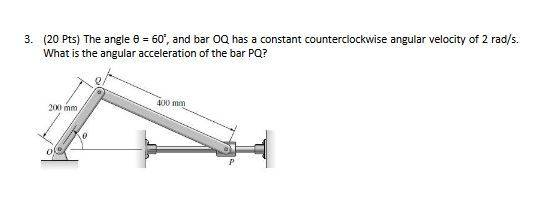# Am I using the Right Formula?

• whitejac

## Homework Statement## Homework Equations

I want to say I should be using this one:
aA = aB + α x rA/B + ω x (ω x rA/B)

## The Attempt at a Solution

The only problem is that if I'm supposed to find the angular acceleration then i'd have to take the... inverse of the cross product? To get it back and I don't think that that's possible. I also want to say that it's zero because OQ moves with constant angular velocity, but I believe that that would result in PQ moving with acceleration because theta would be changing?

I don't think that equation will help.
Just use a bit of geometry to find the angle of the bar PQ as a function of theta.